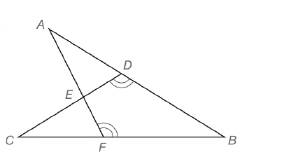Chapter 5.3, Problem 31E### Elementary Geometry for College St...

6th Edition
Daniel C. Alexander + 1 other
ISBN: 9781285195698

#### Solutions

Chapter
Section### Elementary Geometry for College St...

6th Edition
Daniel C. Alexander + 1 other
ISBN: 9781285195698
Textbook Problem
1 views

# Δ A B F ∼ Δ C B D with obtuse angles at vertices D and F as indicated. If m ∠ B = 45 ° , m ∠ C = x and m ∠ A F B = 4 x , find x.Exercises 31, 32

To determine

To find:

The value of x in degree by using the given information.

Explanation

Definition:

CASTC:

Corresponding angles of similar triangles are congruent.

Description:

Given that ΔABFΔCBD with obtuse angles at vertices D and F as indicated. If mB=45°, mC=x and mAFB=4x.

The given figure is shown below.

Figure 1

From the given figure, it is observed that there are two triangles ABF and CBD and which is given that two triangles are similar.

Now draw the two triangles separately as shown below.

Figure 2

Figure 3

Since ΔABFΔCBD and from the definition of CASTC, corresponding angles of similar triangles are congruent

### Still sussing out bartleby?

Check out a sample textbook solution.

See a sample solution

#### The Solution to Your Study Problems

Bartleby provides explanations to thousands of textbook problems written by our experts, many with advanced degrees!

Get Started

#### In Problems 33 – 38, solve each inequality. 38.

Mathematical Applications for the Management, Life, and Social Sciences

#### Which is the best graph of y = f (x) for the given graph of y = f (x)? a) None of these

Study Guide for Stewart's Single Variable Calculus: Early Transcendentals, 8th

#### Define applied research and basic research and identify examples of each.

Research Methods for the Behavioral Sciences (MindTap Course List)